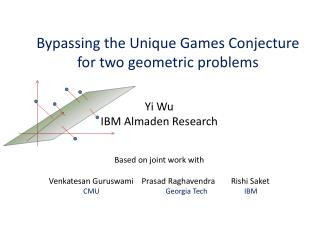Download PresentationBypassing the Unique Games Conjecture for two geometric problems

# Bypassing the Unique Games Conjecture for two geometric problems - PowerPoint PPT Presentation

Bypassing the Unique Games Conjecture for two geometric problems. Yi Wu IBM Almaden Research. Based on joint work with Venkatesan Guruswami Prasad Raghavendra Rishi Saket CMU Georgia Tech IBM . Unique Games Conjecture. Unique Games Conjecture [ Khot 02]I am the owner, or an agent authorized to act on behalf of the owner, of the copyrighted work described.
Download Presentation## Bypassing the Unique Games Conjecture for two geometric problems

Download Policy: Content on the Website is provided to you AS IS for your information and personal use and may not be sold / licensed / shared on other websites without getting consent from its author.While downloading, if for some reason you are not able to download a presentation, the publisher may have deleted the file from their server.

- - - - - - - - - - - - - - - - - - - - - - - - - - E N D - - - - - - - - - - - - - - - - - - - - - - - - - -
Presentation Transcript
1. Bypassing the Unique Games Conjecture for two geometric problems Yi Wu IBM Almaden Research Based on joint work with VenkatesanGuruswami Prasad Raghavendra Rishi Saket CMUGeorgia Tech IBM

2. Unique Games Conjecture • Unique Games Conjecture [Khot 02] • For every there is an integer such that it is NP-hard to decide whether a UG instance on labels has: • (YES instance) • (NO instance)

3. Implications of UGC For a large class of optimization problems, Semidefinite Programming (SDP) gives the best polynomial time approximation. MAX 2SAT MAX 2LIN MAX 2AND Max Cut MAX 3CSP MultiCut 0-EXTENSION Max 3 SAT Multiway Cut MAX 3SAT Max 4 SAT Max 2 SAT

4. Status of the UGC • Lower bound: strong SDP integrality gap instance exists. [KV05, KS09,RS09, BGHMRS] • Upper bound: [Arora-Barak-Steurer 11] can be solved in time . • The reduction from SAT (of size to prove UGC needs to have size blowup if SAT does not have sub-exponential algorithm.

5. Skepticism of UGC • What if UGC is false? The optimality of SDP may not hold. • very few result on the optimality of SDP without UGC. • It is not clear whether Unique Games Conjecture is a necessary assumption for all the hardness results.

6. Overview of our work • For two natural geometric problems, we prove that Semidefinite Programming gives the best polynomial time approximation withoutassuming UGC. • same UG-hardness results known previously.

7. Problem 1: Subspace approximation • Input: a set of points , a number Some constant • Algorithmic task: finding the best dimensional subspace minimize the norm of its Euclidean distance to the points. is the Euclidean distance between and

8. Special case • Objective function: • least square regression. • : Minimum enclosing ball. In this work, we study the problem for

9. Our results where Let be the -th norm of a Gaussian • Previous result: [Deshpande-Tulsiani-Vishnoi11] : • UG hardnessof approximation • approximation by SDP. • Our result: NP hardness of approximation.

10. Problem 2: Quadratic Maximization • Input: a symmetric matrix • Algorithmic goal: Subject to for

11. Special case • : calculating the largest eigenvalue. • : the Grothendieck problem on complete graph. In this work, we study the problem for

12. Previous Result: [Kindler-Naor-Schechtman 06] : • UG hardness • approximation by SDP.

13. Our Result • NP-hardnessof approximation. • approximation by SDP. • independently by [Naor-Schechtman]

14. Remarks on our results • While both problems have nothing to do with Gaussian, involves Gaussian Distribution in a fundamental way. • Gaussian Distribution also occurs fundamentally in UG hard ness proof, coincidence? • Evidence that SDP can be the best algorithm for optimization problems without UGC. • the approximation threshold is : unlikely to have a simple alternative combinatorial algorithm? • Our hardness reduction have size blow up matching the Arora-Barak-Steurer algorithm’s requirement.

15. Proof overview forsubspace approximation

16. Main Gadget: Dictator Test • A instance of subspace approximation over and . Equivalent problem: finding | is the distance from to subspace orthogonal to

17. A Dictator Test instance • Completeness: for every depends only on 1 coordinate (, is less than • Soundness: for every that depends only on a lot of coordinates, is above If we have a dictator test instance, then it is UG-hard get better than-approximation.

18. A -Dictator Test instance • Let be all the points on • (Completeness) When , • (Soundness, informal proof) When by CLT

19. Reduction from Smooth Label Cover Label sets For edge , satisfies if,

20. Smooth Label Cover Theorem [Khot 02] : (soundness), s.t. given an instance with label sets it is NP-hard to decide, OPT() (YES) or OPT() (NO) where satisfies the following property, (smoothness) the set of projections is a good hash family.

21. Rest of the proof • Composing the Smooth Label Cover with the dictator test.

22. Future Work • Other geometric problem with only UG hardness are known. • Kernel Clustering • Learning halfspacesby degree polynomials • Matrix Norm (SSE hardness).